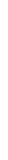# ZK MAPREDUCE

The first product in the Lagrange ZK Big Data stack. Explore how to integrate ZK MapReduce into your DApp.var dataframe = ethereum.createDataFrame({...})

dataframe.select(...)

dataframe.map(...)

dataframe.filter(...)

dataframe.reduce(...)

dataframe.union(...)

dataframe.generateProof({...})

lagrange.submitProof({...})

## GENERATE PROOFS FOR POWERFUL AND EXPRESSIVE Distributed COMPUTATIONS## TREAT STORAGE STATE like A verifiable data lake

Supercharge your dApp with cross-chain and historical storage state. Start with a dataframe, execute MapReduce operations, generate a ZKP, and verify on chain.//Create a dataframe of storage proofs for 1024 consecutive blocks of Polygon

var dataframe = polygon.createDataFrame({
block_range_start:polygon.currentBlock() - 1024,
block_range_start:polygon.currentBlock(),
interval: 1,
content:{
keys:[
{name: liquidity_1, memory:"0x56e81...21"},
{name: liquidity_2, memory:"0x56211...32"},
]
}
})

//Create a dataframe of storage proofs for 1024 consecutive blocks of Polygon

var dataframe = polygon.createDataFrame({
block_range_start:polygon.
currentBlock() - 1024,
block_range_start:polygon.
currentBlock(),
interval: 1,
content:{
keys:[
{name: liquidity_1,
memory:"0x56e81...21"},
{name: liquidity_2,
memory:"0x56211...32"},
]
}
})//Compute block-by-block price for each asset pair (a map step)

var dataframe = polygon.map("asset_price",     polygon.liquidity_1.

div(polygon.liquidity_2))

//Compute Standard Deviation (a reduce step)

var price_mean =
polygon.reduce(avg("asset_price"))

//Compute Mean (a reduce step)

var price_std =
polygon.reduce(stddev(
"asset_price"))

//Filter outliers using standard deviation

dataframe = polygon.filter(
polygon.asset_price.lt(mean - 2 * price_std) ||
polygon.asset_price.lt(mean + 2 * price_std))

//Compute mean without outliers

var outlier_resistant_mean =
polygon.reduce(avg("asset_price"))## ZK Map Reduce MAKES MERGING CROSS-CHAIN STORAGE PROOFS A Breeze

Configure your chains, contract addresses and storage slots. Generate batch storage proofs with dynamic distributed computation.

ZKMR lets developers build expressive and data rich dApps with multi-chain and historical states, secured with zero-knowledge proofs.//Create a dataframe of storage proofs for 1024 consecutive blocks of Optimism

var dataframe_Optimism = optimism.createDataFrame({
block_range_start:optimism.
currentBlock() - 1024,
block_range_start:optimism.
currentBlock(),
interval: 1,
content:{
block_hash:"0xd1c9...3d",
keys:[
{name: liquidity_1,
memory:"0x12a50...16"},
{name: liquidity_2,
memory:"0x12a50...17"},
]
}
})

//Compute block-by-block price for each asset pair (a map step) for Polygon and Optimism dataframes

dataframe_Poly = dataframe_Poly.map("asset_price",
dataframe_Poly.liquidity_1.
div(dataframe_Poly.liquidity_2))
dataframe_Opt = dataframe_Opt.map("asset_price",
dataframe_Opt.liquidity_1.
div(dataframe_Opt.liquidity_2))

//Union Polygon and Optimism dataframes and compute mean price

var dataframe_Merged = dataframe_Poly.union(dataframe_Opt)

dataframe_Merged.reduce(
avg("asset_price"))

//Note: For chains with different block times, a user should normalize for price * time

//Create a dataframe of storage proofs for 1024 consecutive blocks of Optimism

var dataframe_Optimism = optimism.createDataFrame({
block_range_start:optimism.
currentBlock() - 1024,
block_range_start:optimism.
currentBlock(),
interval: 1,
content:{
block_hash:"0xd1c9...3d",
keys:[
{name: liquidity_1, memory:"0x12a50...16"},
{name: liquidity_2, memory:"0x12a50...17"},
]
}
})

//Compute block-by-block price for each asset pair (a map step) for Polygon and Optimism dataframes

dataframe_Poly = dataframe_Poly.map("asset_price",
dataframe_Poly.liquidity_1.
div(dataframe_Poly.liquidity_2))
dataframe_Opt = dataframe_Opt.map("asset_price",
dataframe_Opt.liquidity_1.
div(dataframe_Opt.liquidity_2))

//Union Polygon and Optimism dataframes and compute mean price

var dataframe_Merged = dataframe_Poly.union(dataframe_Opt)

dataframe_Merged.reduce(
avg("asset_price"))

//Note: For chains with different block times, a user should normalize for price * time

## Verifiable On-chain STATE

Easily verify computation on any chain directly from your smart contracts. Let your contracts have full access to all historical and cross-chain states.

//Generate ZKMR Proof

//Submit proof to chain

lagrange.submitProof({
chain: "ethereum",
provider: rpc_provider,
publicStatement: dataframe_Merged.publicStatement,
proof: dataframe_Merged.proof
})

//Generate ZKMR Proof

dataframe_Merged.generateProof({
location: "remote",

//Submit proof to chain

lagrange.submitProof({
chain: "ethereum",
provider: rpc_provider,
publicStatement: dataframe_Merged.publicStatement,
proof: dataframe_Merged.proof
})